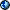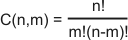Historical Archive of the Bible Wheel Site

The Bible Wheel has been debunked by its author.
Read all about it: Debunking Myself: What A Long Strange Trip It's Been

Recent Blog Articles

# Probablity of the Old Testament Symmetry

Judge not according to the appearance, but judge righteous judgment.

- John 7:24

In a thread called The Canonization of Maccabeeson www.ChristianForums.comI argued for the traditional 39 Book Old Testament Canon by asserting that the astounding three levels of symmetry of the Old Testament is evidence of divine design. The three levels are:

Level One Symmetry: 17 : 5 : 17 17 Books of History, 5 Books of Wisdom and Poetry, 17 of Books of Prophecy.

Level Two Symmetry: 5 : 12 : 5 : 5 : 12 The pattern above subdivides into 5 Books of the Torah, 12 Books of OT History, 5 Books of Wisdom and Poetry, 5 Books of the Major Prophets, 12 Books of the Minor Prophets.

Level Three Symmetry: 5 : 9 : 3 : 5 : 5 : 9 : 3 The pattern above further subdivides into 5 Books of the Torah, 9 Books of Pre-Exilic History, 3 Books of Post-Exilic History, 5 Books of Wisdom and Poetry, 5 Books of the Major Prophets, 9 Books of the Pre-Exilic Minor Prophets, 3 Books of the Post-Exilic Minor Prophets.

After displaying this pattern I asserted that it was a "strong sign of design." A member named "Philip" challenged my assertion by writing:

Do you have a basis for this claim? Statistical theory suggests exactly the opposite. In any structure as complex as the OT, the chance of there not being some local organisation are astronomically small.

Contrary to Philip's assertion, statistical theory shows that the organization of the Old Testament as we have it in the Protestant Bible is extremely unlikely to have occured by chance. There is exactly one chance in 48,433 that the pattern would appear by accident.

The math required to prove this is elementary. Consider a list of 39 objects. How many ways is there to divide them into three groups? The answer is easy to calculate. Begin by forming an abritrary division by dropping two "dividers" | anywhere in the list below.

1 2 3 4 | 5 6 7 8 9 10 11 ... 35 | 36 37 38 39

How many ways can we do this? Well, there are 38 slots to drop the first divider, and then there will be 37 slots to drop the second divider, so there are 38 x 37 = 1406 ways to drop the dividers between 39 books. But some of But we also need to divide by the number of ways to arrange the dividers, since interchanging them will lead to an identical division. The final answer is given by the Binomial Coefficients, also known as "Choice Numbers", where "n factorial" is given by n! = n x n-1 x n-2 x ... x 3 x 2 x 1:Thus there are C(38,2) = (38 x 37)/(2 x 1) = 1406/2 = 703 ways to divide 39 Books into three groups. This is basic Combinatorics. I used similar calculations to determine the probability of the sevenfold symmetry of the whole Bible [see Probabilities - What are the Chances?].

And how many of these will show the Level One Symmetry like the 17 : 5 : 17 of the OT? That too is easy to calculate. Suppose the first divider is dropped between books n and n+1. To achieve the symmetry, the second divider must be dropped between books 39-n and 39-n+1. Thus we will have the symmetry of n : 39-2n : n. Since n can range over 1 to 19, we have exactly 19 possible ways to divide the 39 books into three groups that have the same Level One Symmetry as the OT.

So what is the probability that we would find a canon with the Level One Symmetry of the OT "by chance"? It is simply the ratio 19/703 = 1/37. Not too rare really. The Level One Symmetry by itself is not proof of design.

But now lets consider the second level of symmetry. We do the same calculation as above, only now we drop in to more dividers:

C(38,4) = 38!/(34!)(4!) = 73,815 ways to divide 39 books into 5 goups.

How many of these will show the Level Two Symmetry of the OT? To see this, we need to write it abstactly as follows:

Level One: n : 39-2n : n [OT has n=17 to give 17 : 5 : 17]

Level Two: n-m : m : 39-2n : n-m : m [OT has n=17, m=12 to give 5 : 12 : 5 : 5 : 12 : 5]

Now to achieve a symmetry for all m like the second level of the OT, the size of the first, the third, and the fourth divisions must all the same. In the existing Canon, these groups all have 5 Books. Mathematically, this demands that n-m = 39-2n. This gives a restraint on both m and n:

m = 3n-39, and since 0 < m < n-1, we also have a restriction on n such that 13 < n < 20.

 All Possible Level TwoSymmetric Canons n=14 11 : 3 : 11 : 11 : 3 n=15 9 : 6 : 9 : 9 : 6 n=16 7 : 9 : 7 : 7 : 9 n=17 5 : 12 : 5 : 5 : 12 n=18 3 : 15 : 3 : 3 : 15 n=19 1 : 18 : 1 : 1 : 18

Putting this all together, we see that the Level Two Symmetry demands a pattern that can be written in terms of a single variable "n" that ranges from 14 to 19:

39-2n : 3n-39 : 39-2n : 39-2n : 3n-39 with 13 < n < 20

As it turns out, there are only six solutions! They are listed in the table. This means that out of the 73,815 possible divisions of 39 Books into seven divisions, there are only six that show the Level Two Symmetry that we see in the Canon God designed. That's exactly one chance in 12,302.5 (= 6/73,815) that the symmetric structure of the Old Testament appeared by accident. The numbers now are beginning to look quite persuasive, and this is only at the second level of symmetry.

But there's another thing to consider before moving on to the third level. I speak of the self-witness of Scritpure to the meaning of the numbers God used in the design of the Old Testament. How many loaves fed the five thousand? FIVE. How many baskets left over? TWELVE. What is the symbolic meaning of BREAD in the Bible? It is the WORD OF GOD that feeds the disciples of Christ. The symbolic meanings of the numbers God used in the design of His Word are explained in the text of His Word!

Moving on to the third level of symmetry, we do the same math as above and drop in two more divisions:

C(38,6) =38!/(32!)(6!) = 2,760,681 ways to divide 39 books into 7 goups.

That's over 2.7 MILLION ways to divide 39 books into seven divisions. And how many of these will show the Level Three Symmetry that we see in the OT? That's easy to calculate. The third level of symmetry is found by dividing the second and fifth divisions in the same way. These divisions are restricted to 3, 6, 9, 12, 15 or 18 Books by the Level Two Symmetry shown in the table above. The third level of symmetry is abstractly represented as follows, where 3n-39-k and k represents the size of the two new subdivisions:

39-2n : 3n-39-k : k: 39-2n : 39-2n : 3n-39-k : k with 0 < k < 3n-39

 All Possible Level Three Symmetric Canons n=14 11 : 3-k : k : 11 : 11 : 3-k : k 0 < k < 3 2 Solutions n=15 9 : 6-k : k : 9 : 9 : 6-k : k 0 < k < 6 5 Solutions n=16 7 : 9-k : k : 7 : 7 : 9-k : k 0 < k < 9 8 Solutions n=17 5 : 12-k : k : 5 : 5 : 12-k : k 0 < k < 12 11 Solutions n=18 3 : 15-k : k : 3 : 3 : 15-k : k 0 < k < 15 14 Solutions n=19 1 : 18-k : k : 1 : 1 : 18-k : k 0 < k < 18 17 Solutions

There are 3n-39 solutions for each of the six Level Two solutions listed above. The total number of Level Three solutions is simply the sum of these. I've listed all possible solutions in the table to avoid too much abstract mathematics for those who are not familiar with it.

Summing the last column shows that there are exactly 57 canonical structures with a Level Three Symmetry out of a total of 2,760,681 possible ways to divide the 39 Books. That is one chance in 48,433 that the Level Three Symmetry of the Protestant Old Testament Canon happened by accident. The overwhelming majority of possible 39 Book canons would not exhibit a Three Level Symmetry like the Canon God designed. And remember, all this is happening in a Book that claims to be inspired from God! The proof is both final and complete.

Yet there is still more compelling evidence. There is another witness from the text of Scripture itself. The two divisions that have 17 Books are each divided into 14 and 3 with the Babylonian Exile punctuating the division in both the Historical and the Prophetical Books! This reflects the pattern of the geneology of the Lord Jesus Christ, which is also punctuated by the Babylonian Exile and the Number 14 (Matt 1:17):

So all the generations from Abraham to David are fourteen generations;
and from David until the carrying away into Babylon are fourteen generations;
and from the carrying away into Babylon unto Christ are fourteen generations.

This "coincidence" evokes a mystical sense of the whole Bible as the "book of the generation (lit. genesis) of Jesus Christ" [see A Great Clound of Witnesses].